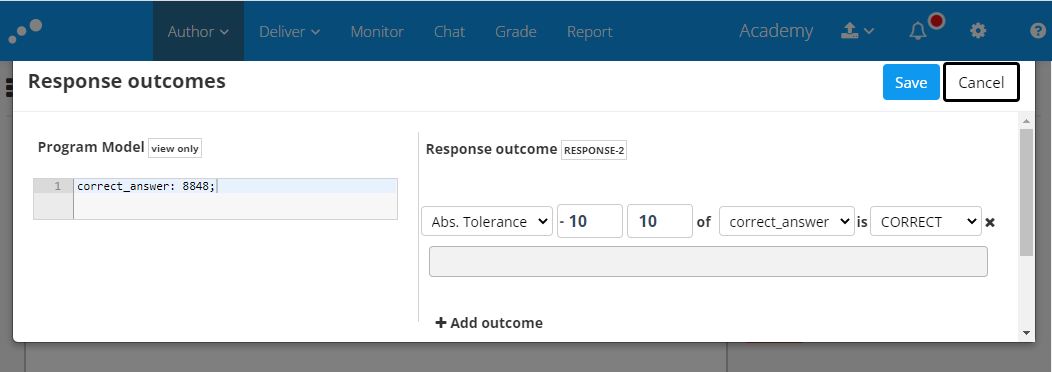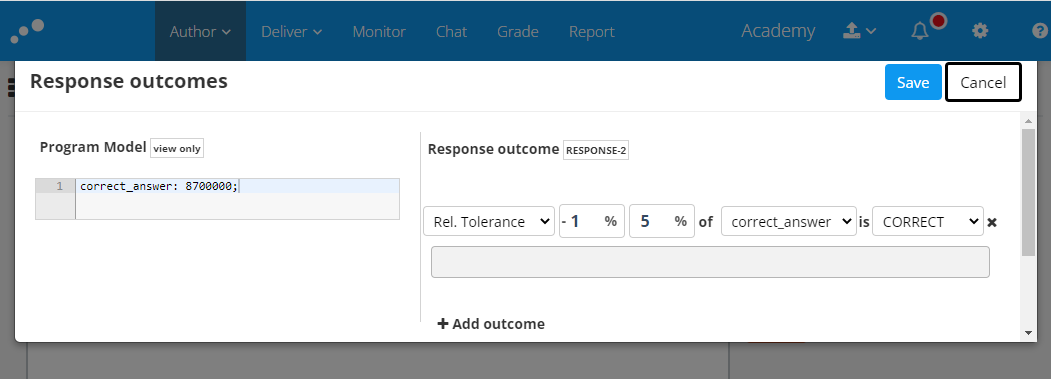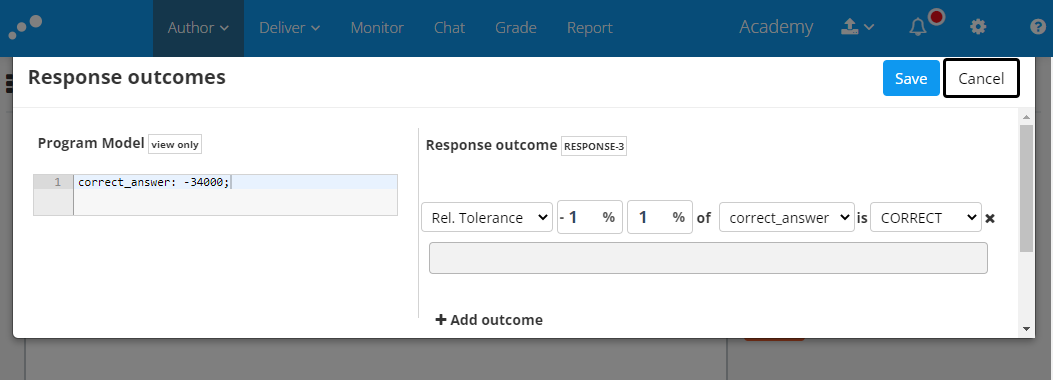# Numerical simulation - Absolute and Relative tolerance

This is available for Authors.

When creating questions in Numerical Simulation, you have the ability to define tolerance levels for the deviation of results. This feature allows you to author more comprehensive questions while ensuring that candidates are not penalized for small deviations that still demonstrate their understanding.

To maintain compatibility with the QTI (Question and Test Interoperability) standard for storing content and enabling the use of these questions in other systems, we utilize the formulas defined by the standard for evaluating tolerance levels (found below).

# Absolute tolerance

Absolute tolerance is used when you want to define a response outcome that falls within an absolute lower and upper bound of a variable. For a candidate response to meet the response outcome, it should be within the range of [variable]-lower bound, [variable]+upper bound, where lower and upper bounds are positive numbers.

## ExampleQuestion:

How high is Mount Everest?

Instructions:

I define the variable for the correct answer to be 8848 (meters) and want to allow +/- 10 meters to allow for fluctuations in snow levels.

Adding 10 as both lower and upper bounds, and setting the response outcome as CORRECT, will evaluate answers within the range 8838, 8858 as CORRECT (calculated by inserting the numbers into the formula above: 8848-10, 8848+10).

# Relative tolerance

Relative tolerance allows you to define a response outcome that falls within a specified percentage range of a variable, rather than requiring an exact match. The acceptable range is determined by [variable]*(1-lower bound/100), [variable]*(1+upper bound/100), with both the lower and upper bound as positive numbers.

Note: When using negative values and calculating relative tolerance, it is important to verify the desired outcome by plugging the numbers into the provided formula.

## Example 1Question:

What is the population of Switzerland?

Instructions:

The right answer is around 8,7 million. Due to fluctuations, we want to allow for a lower bound of 1% deviation and upper bound of 5% deviation.

Adding 1 as lower bound and 5 as upper bound, and setting the response outcome as CORRECT, will evaluate answers within the range 8,613 million and 9,135 million as CORRECT. This is calculated by inserting the numbers into the formula above: 8700000*(1-1/100), 8700000*(1+5/100).

Note: care should be taken when working with negative values. When calculating the relative tolerance from a negative number, remember to verify that you get the desired outcome by inserting your numbers into the formula above.

## Example 2Question:

How much did Japan’s birth population decline in 2020 compared to 2019? Express your answer as a negative number.

Instructions:

The right answer is around -34,000. Due to fluctuations, we want to allow for a lower bound of 1% deviation and upper bound of 1% deviation.

Adding 1 as lower bound and 1 as upper bound, and setting the response outcome as CORRECT, will evaluate answers within the range -33,660 and -34,340 as CORRECT. This is calculated by inserting the numbers into the formula above:-34,000*(1-1/100), -34,000*(1+1/100).